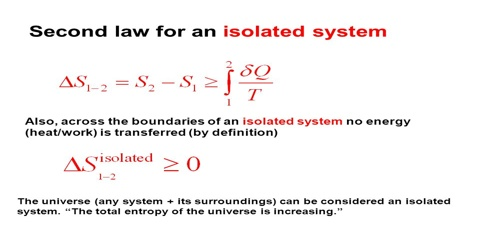# Total Entropy Change of a System and its Surroundings

Total entropy change of a system and its surroundings

A system is that part which is under investigation. The surroundings constitute all other parts which might interact with the system. In most cases, the surroundings consist of heat reservoirs that can add heat to or take out heat from the system or of a mechanical device which does work on or accept work from a system.

In a reversible process, when an amount dqrev of heat is absorbed by the system an equal amount is lost by the surroundings. The change in entropy of the system is equal and opposite to the change in entropy of the surroundings, so that the total changes in entropy of the system and the surroundings is zero.

Consider now, an irreversible process in which the system absorbs an amount dqirrev of heat and on from state A to state B. Entropy being a state function, the gain in entropy of the system is given by SB – SA. This gain in entropy, of course, is calculated from the relation:

∆S = SB – SA = BA (qrev/T)

where dqrev is the heat that the system would have absorbed if the process were carried out reversibly. During the process the surroundings have supplied a quantity of heat dqirrev. Although this heat has been taken up irreversibly by the system, the change in entropy of the surroundings is calculated by supposing that this quantity of heat has been given out reversibly. Hence the decrease in entropy of the surroundings is given by BA (qrev/T). The total change of entropy of the system and the surroundings when the system goes from state A to state B will he obtained in the following way:

Gain in entropy of the system = SB – SA

Loss in entropy of the surrounding = BA (qrev/T)

Net change in entropy = (SB – SA) – BA (qrev/T)

According to the relation, the value of the integral is always less than SB – SA, so that the net change in entropy is a positive quantity; that is, as a result of the process there is a gain in entropy of the system and the surroundings taken together. Hence, if any part of a process is irreversible there is an increase in entropy when the system and its surroundings are considered together. Natural processes are spontaneous and therefore, irreversible. It follows, then, that natural processes are accompanied by an increase in entropy and the sum total of entropy in the universe is increasing. This deduction led Clausius to make his famous statement of the laws of thermodynamics:

“The energy of the universe is constant; the entropy of the universe tends always towards a maximum.”

This conclusion from the second law of thermodynamics has profound philosophical implications. Considering the system and the surrounding together we can, in summary, write:

∆S = 0 for a reversible process

and ∆S > for a natural process